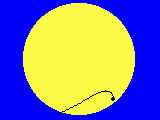Essen, horizontal view

# Transit of Venus, June 8th, 2004Essen, equatorial view

## Project 1: Measuring the Radius of Venus' Orbit

To be able to derive an own measure of the Astronomical Unit from the self determined value of the Sun's parallax by the following equation (see the related paper (in German or in English))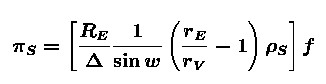you need to determine, among other quantities, the radius rV of Venus' orbit or, to be more precise, the relation rV /rE between the radii of the orbits of Venus and the Earth.

There are several possibilities for this measurement:

1. Measuring the maximum angular distance between Sun and Venus both of them above the horizont at the same time by means of a simple device for angle measurements or, alternatively, with a sextant.

2. Other methods of determing the maximum angular distance between Sun and Venus, for instance

1. observing Venus at the night sky, recognizing her position relative to stars in the neighborhood and determing her equatorial position by drawing her into a star map and
2. measuring the exact horizontal position of the Sun and transforming her horizontal to equatorial coordinates. (For this method, you need to know how to determine the local sideral time and your own geographical position.)

3. Observing Venus during her retrograde motion and measuring her equatorial positon at least two times.
All of these methods make use of the following simplifications:
• All planets move in the same plane as the Earth.
• All orbits are circles with the Sun in their center.
• All planets move with constant speed.

### 1. Direct measurement of the maximum angular distance between Sun and Venus

When the angular distance between Sun and Venus, observed from Earth, has its maximum value the triangle Sun - Venus - Earth is rectangular.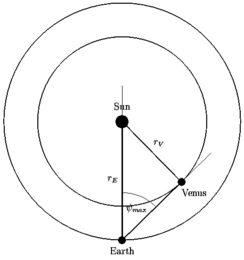Therefore, the radius of Venus' orbit, in Astronomical Units, can easily be determined by measuring the maximum angular distance between Sun and Venus:Because Venus will reach the maximum angular distance to the Sun around the end of March 2004 the suitable time for doing these measurements are the weeks of March and April 2004! In these weeks, Venus will play the role of the evening star so that the measurements can be done in the evening.
The following possibility will occur after the transit in August. Than, Venus will act as morning star.

The simplest method to measure the distance and to determine its maximum is to observe both objects above the horizont, simultaneously. If you observe Venus at the night sky early in the morning you will be able to find her shortly after sunrise.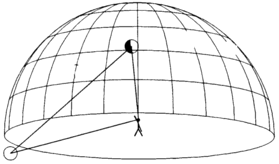Proposals for rough measurements of the angle: (You may get an impression of the measurements on our first result page.)

• Mark the lines of sight with pins on a piece of carton.
• Use a pair of compasses.
Perhaps you will find other simple possibilities.

A more precise method is to use a sextant a device which was invented just for this kind of measurements.### 2. Measuring the equatorial positions of Venus and the Sun

This method is more precise than that described above. But it is more indirect and you need more knowledge (about celestial coordinates and sideral time, for instance) and more mathematical abilities (transformation of coordinates and so on).

#### 2.1 Position of the Sun

The simplest method of measuring the equatorial position of the Sun is to measure the exact time of its culmination and its maximum elevation. Then, there holds a simple relation between declination of the Sun, geographical latitude of the observer and the maximum elevation, for instance with a gnomon (see also project 2):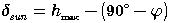(for northern latitude)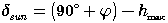(for southern latitude)

The right ascension of the Sun is just the exact local sideral time of the time of culmination.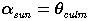#### 2.2 Position of Venus

The easiest method of determing the celestial position of Venus is to observe her exact position among the stars before sunrise and draw her into a sky map from which you can read the equatorial coordinates.Position of Venus and Mars on January 11th, 2003

#### 2.3 Evaluating the horizontal position of Venus and Sun

For this method you have to measure the azimut A, for instance with a magnetic compass, and the elevation h above the horizont and the exact time of your measurement. From this values, you can derive the equatorial coordinates by the following algorithm: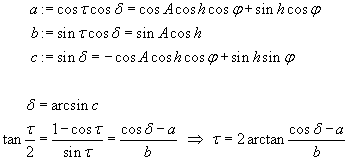From the hour angle, you can derive the right ascension when you know the local sideral time: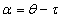#### 2.4 Calculating the angular distance

If you know the equatorial positions of Venus and the Sun it is not difficult to calculate their angular distance by the following formular (see below):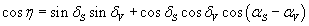### 3. Observing Venus during her retrograde motion

This method can be applied only in a short time interval around the transit, that is between May 23th and June 28th!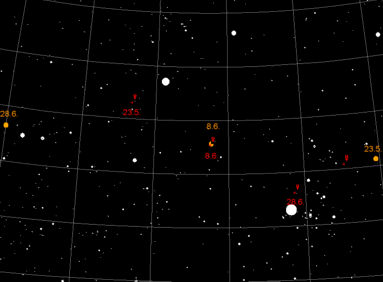Venus' positions at the beginning and at the end of its retrograde motion

Around the inferior conjunction of Venus, at the sky the planet will move retrogradely that means opposite to its usual direction (from east to west). That's why Venus overtakes the Earth in that time. The picture below gives an explanation for this fact not only in a qualitative way but in a quantitative, too.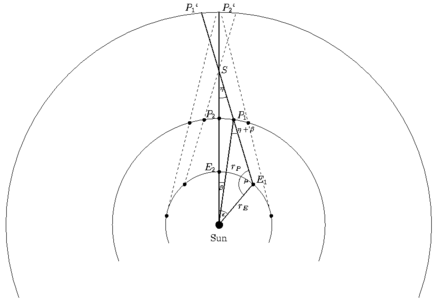Explanation of the retrograde motion of a superior planet. In the case of Venus which is an inferior planet you must change the letters P and E. The argumentation remains unaltered.

In the triangle Sun - E1 - P1, the following equation holds: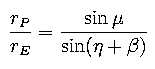Looking at the triangle Sun - E1 - S you may realize the following identity:Combining the both equations, we get the following result: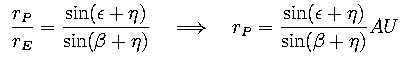We hope that we will be able to observe and to measure Venus's position in its inferior conjunction, that is during its transit! Thus, we can measure its right ascension and declination on that day (see project 4). If we will be able to measure Venus' position on an additional day during its retrograde motion we can determine the anglular distance η between these both positions by the following relation: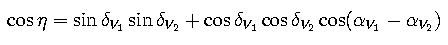The central angles at the Sun can be derived from the mean daily motion of the planets which are due to their sideral periods:### Generalization

If we will be unlucky and not able to observe the transit we, nevertheless, can apply the above method by measuring Venus' position during its retrograde motion twice. In this case, the following equation must hold: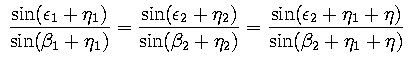In this equation, the single angles η1 and η2 are unknown. Only the angle η=η21 has been measured. The central angles are again determined with respect to the date of conjunction.

The following function f must, therefore, be zero at the point η1: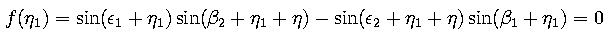This is a well known numerical problem and can be solved by trial and error, by a graphical method or by a numerical algorithm, respectively. You may download a little program which does the work for you.

### 4. First Results

Groups in Fürstenwalde und Essen have determined the radius of Venus' orbit between the end of December 2002 and the end of January 2003. The results are shortly combined in a result page.

The mean value of these measurements is:Prof. Dr. Udo Backhaus
last modification:  March 28th, 2008